Community Articles
Find and share helpful community-sourced technical articles.

# Spark RDDs vs DataFrames vs SparkSQL

Labels (1)
• ### Apache Sparkbmathew
Guru

## Synopsis

This tutorial will demonstrate using Spark for data processing operations on a large set of data consisting of pipe delimited text files. Delimited text files are a common format seen in Data Warehousing:

1. Random lookup for a single record
2. Grouping data with aggregation and sorting the output

3 Different techniques will be used to solve the above 2 problems and then compare how they perform against each other:

1. Using RDD’s
2. Using DataFrames
3. Using SparkSQL

The purpose of this tutorial is to provide you with code snippets for the above 3 techniques and to demonstrate how RDD’s outperform DataFrames and SparkSQL for certain types of data processing. See below at the end of this article for all code.

Also, these tests are demonstrating the native functionality within Spark for RDDs, DataFrames, and SparkSQL without calling additional modules/readers for file format conversions or other optimizations.

For joining datasets, DataFrames and SparkSQL are much more intuitive to use, especially SparkSQL, and may perhaps yield better performance results than RDDs.

## Source Data:

• 9 Million unique order records across 3 files in HDFS
• Total size of HDFS data files is 1.4 GB
• Each order record could be for 1 of 8 different products
• Pipe delimited text files with each record containing 11 fields
• Data is fictitious and was auto-generated programmatically

• HDP 2.4
• Spark 1.6
• HDP Sandbox

## RDDs, DataFrames, and SparkSQL:

At its core, Spark operates on the concept of Resilient Distributed Datasets, or RDD’s:

• Resilient - if data in memory is lost, it can be recreated
• Distributed - immutable distributed collection of objects in memory partitioned across many data nodes in a cluster
• Dataset - initial data can from from files, be created programmatically, from data in memory, or from another RDD

DataFrames API is a data abstraction framework that organizes your data into named columns:

• Create a schema for the data
• Conceptually equivalent to a table in a relational database
• Can be constructed from many sources including structured data files, tables in Hive, external databases, or existing RDDs
• Provides a relational view of the data for easy SQL like data manipulations and aggregations
• Under the hood, it is a row of RDD’s

SparkSQL is a Spark module for structured data processing. You can interact with SparkSQL through:

• SQL
• DataFrames API
• Datasets API

## Test results:

• RDD’s outperformed DataFrames and SparkSQL for certain types of data processing
• DataFrames and SparkSQL performed almost about the same, although with analysis involving aggregation and sorting SparkSQL had a slight advantage
• Syntactically speaking, DataFrames and SparkSQL are much more intuitive than using RDD’s
• Took the best out of 3 for each test
• Times were consistent and not much variation between tests
• Jobs were run individually with no other jobs running
1. Random lookup against 1 order ID from 9 Million unique order ID's
2. GROUP all the different products with their total COUNTS and SORT DESCENDING by product name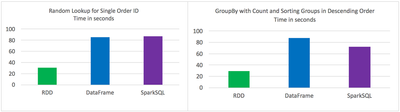## Code:

RDD Random Lookup

```#!/usr/bin/env python

from time import time
from pyspark import SparkConf, SparkContext

conf = (SparkConf()
.setAppName("rdd_random_lookup")
.set("spark.executor.instances", "10")
.set("spark.executor.cores", 2)
.set("spark.dynamicAllocation.enabled", "false")
.set("spark.shuffle.service.enabled", "false")
.set("spark.executor.memory", "500MB"))
sc = SparkContext(conf = conf)

t0 = time()

path = "/data/customer_orders*"
lines = sc.textFile(path)

## filter where the order_id, the second field, is equal to 96922894
print lines.map(lambda line: line.split('|')).filter(lambda line: int(line) == 96922894).collect()

tt = str(time() - t0)
print "RDD lookup performed in " + tt + " seconds"```

DataFrame Random Lookup

```#!/usr/bin/env python

from time import time
from pyspark.sql import *
from pyspark import SparkConf, SparkContext

conf = (SparkConf()
.setAppName("data_frame_random_lookup")
.set("spark.executor.instances", "10")
.set("spark.executor.cores", 2)
.set("spark.dynamicAllocation.enabled", "false")
.set("spark.shuffle.service.enabled", "false")
.set("spark.executor.memory", "500MB"))
sc = SparkContext(conf = conf)

sqlContext = SQLContext(sc)

t0 = time()

path = "/data/customer_orders*"
lines = sc.textFile(path)

## create data frame
orders_df = sqlContext.createDataFrame( lines.map(lambda l: l.split("|")) .map(lambda p: Row(cust_id=int(p), order_id=int(p), email_hash=p, ssn_hash=p, product_id=int(p), product_desc=p, country=p, state=p, shipping_carrier=p, shipping_type=p, shipping_class=p  ) ) )

## filter where the order_id, the second field, is equal to 96922894
orders_df.where(orders_df['order_id'] == 96922894).show()

tt = str(time() - t0)
print "DataFrame performed in " + tt + " seconds"```

SparkSQL Random Lookup

```#!/usr/bin/env python

from time import time
from pyspark.sql import *
from pyspark import SparkConf, SparkContext

conf = (SparkConf()
.setAppName("spark_sql_random_lookup")
.set("spark.executor.instances", "10")
.set("spark.executor.cores", 2)
.set("spark.dynamicAllocation.enabled", "false")
.set("spark.shuffle.service.enabled", "false")
.set("spark.executor.memory", "500MB"))
sc = SparkContext(conf = conf)

sqlContext = SQLContext(sc)

t0 = time()

path = "/data/customer_orders*"
lines = sc.textFile(path)

## create data frame
orders_df = sqlContext.createDataFrame( lines.map(lambda l: l.split("|")) .map(lambda p: Row(cust_id=int(p), order_id=int(p), email_hash=p, ssn_hash=p, product_id=int(p), product_desc=p, country=p, state=p, shipping_carrier=p, shipping_type=p, shipping_class=p  ) ) )

## register data frame as a temporary table
orders_df.registerTempTable("orders")

## filter where the customer_id, the first field, is equal to 96922894
print sqlContext.sql("SELECT * FROM orders where order_id = 96922894").collect()

tt = str(time() - t0)
print "SparkSQL performed in " + tt + " seconds"```

RDD with GroupBy, Count, and Sort Descending

```#!/usr/bin/env python

from time import time
from pyspark import SparkConf, SparkContext

conf = (SparkConf()
.setAppName("rdd_aggregation_and_sort")
.set("spark.executor.instances", "10")
.set("spark.executor.cores", 2)
.set("spark.dynamicAllocation.enabled", "false")
.set("spark.shuffle.service.enabled", "false")
.set("spark.executor.memory", "500MB"))
sc = SparkContext(conf = conf)

t0 = time()

path = "/data/customer_orders*"
lines = sc.textFile(path)

counts = lines.map(lambda line: line.split('|')) .map(lambda x: (x, 1)) .reduceByKey(lambda a, b: a + b) .map(lambda x:(x,x)) .sortByKey(ascending=False)

for x in counts.collect():
print x + '\t' + str(x)

tt = str(time() - t0)
print "RDD GroupBy performed in " + tt + " seconds"```

DataFrame with GroupBy, Count, and Sort Descending

```#!/usr/bin/env python

from time import time
from pyspark.sql import *
from pyspark import SparkConf, SparkContext

conf = (SparkConf()
.setAppName("data_frame_aggregation_and_sort")
.set("spark.executor.instances", "10")
.set("spark.executor.cores", 2)
.set("spark.dynamicAllocation.enabled", "false")
.set("spark.shuffle.service.enabled", "false")
.set("spark.executor.memory", "500MB"))
sc = SparkContext(conf = conf)

sqlContext = SQLContext(sc)

t0 = time()

path = "/data/customer_orders*"
lines = sc.textFile(path)

## create data frame
orders_df = sqlContext.createDataFrame( lines.map(lambda l: l.split("|")) .map(lambda p: Row(cust_id=int(p), order_id=int(p), email_hash=p, ssn_hash=p, product_id=int(p), product_desc=p, country=p, state=p, shipping_carrier=p, shipping_type=p, shipping_class=p  ) ) )

results = orders_df.groupBy(orders_df['product_desc']).count().sort("count",ascending=False)

for x in results.collect():
print x

tt = str(time() - t0)
print "DataFrame performed in " + tt + " seconds"```

SparkSQL with GroupBy, Count, and Sort Descending

```#!/usr/bin/env python

from time import time
from pyspark.sql import *
from pyspark import SparkConf, SparkContext

conf = (SparkConf()
.setAppName("spark_sql_aggregation_and_sort")
.set("spark.executor.instances", "10")
.set("spark.executor.cores", 2)
.set("spark.dynamicAllocation.enabled", "false")
.set("spark.shuffle.service.enabled", "false")
.set("spark.executor.memory", "500MB"))
sc = SparkContext(conf = conf)

sqlContext = SQLContext(sc)

t0 = time()

path = "/data/customer_orders*"
lines = sc.textFile(path)

## create data frame
orders_df = sqlContext.createDataFrame(lines.map(lambda l: l.split("|")) .map(lambda r: Row(product=r)))

## register data frame as a temporary table
orders_df.registerTempTable("orders")

results = sqlContext.sql("SELECT product, count(*) AS total_count FROM orders GROUP BY product ORDER BY total_count DESC")

for x in results.collect():
print x

tt = str(time() - t0)
print "SparkSQL performed in " + tt + " seconds"```

## Verification:

Source data files in HDFS: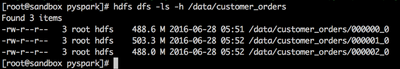Total size of source data files in HDFS: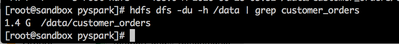Order ID is second field in pipe delimited file. There are 9 Million unique order ID records: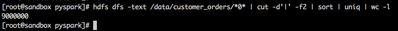Output produced by GroupBy, Count, and Sort Descending (format will not be same for all, however, numbers will be same):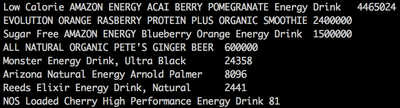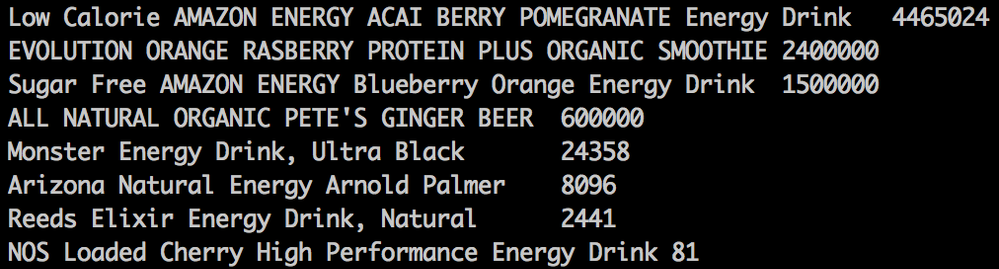81,741 Views

# Re: Spark RDDs vs DataFrames vs SparkSQLmgaido
Rising Star

Very interesting, but I think it'd have been as more even comparision if you'd have used SparkSQL csv reader from databricks to read the file for DataFrame and SparkSQL tests, otherwise there is the overhead of converting the RDD to a DataFrame...

# Re: Spark RDDs vs DataFrames vs SparkSQL

Not applicable

can we say this difference is only due to the conversion from RDD to dataframe ?

because as per apache documentation, dataframe has memory and query optimizer which should outstand RDD

I believe if the source is json file, we can directly read into dataframe and it would definitely have good performance compared to RDD

and why Sparksql has good performance compared to dataframe for grouping test ? dataframe and sparkSQL should be converted to similare RDD code and has same optimizers

# Re: Spark RDDs vs DataFrames vs SparkSQLdhananjay_patka
New Contributor

Is this still valid? I mean there are many improvements on spark-sql & catalyst engine since spark 1.6.

# Re: Spark RDDs vs DataFrames vs SparkSQLroise0r
New Member

Is the input dataset available somewhere?

Take a Tour of the Community
Don't have an account?